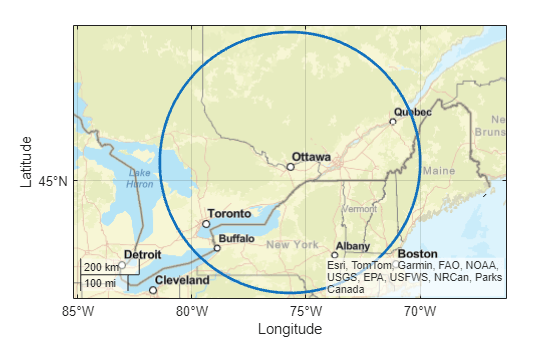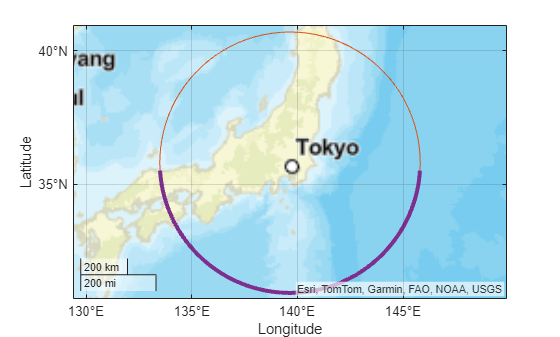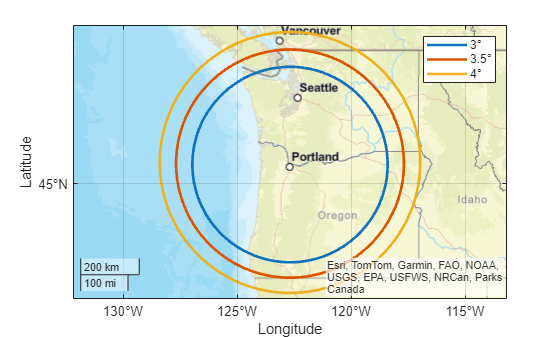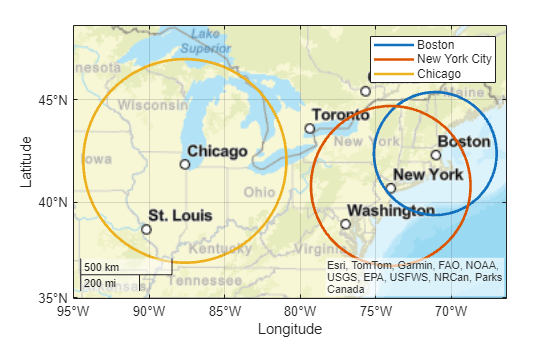# scircle1

Small circle from center and radius

## Syntax

``[lat,lon] = scircle1(lat0,lon0,r)``
``[lat,lon] = scircle1(lat0,lon0,r,az)``
``[lat,lon] = scircle1(lat0,lon0,r,az,ellipsoid)``
``[lat,lon] = scircle1(___,units)``
``[lat,lon] = scircle1(lat0,lon0,r,az,ellipsoid,units,npts)``
``[lat,lon] = scircle1(method,___)``
``mat = scircle1(___)``

## Description

example

````[lat,lon] = scircle1(lat0,lon0,r)` finds the latitude and longitude coordinates of a small circle. Specify the geographic coordinates of the center of the small circle using `lat0` and `lon0`. Specify the radius of the small circle using `r`. This syntax assumes that the radius is a spherical distance in degrees.```

example

````[lat,lon] = scircle1(lat0,lon0,r,az)` finds coordinates for the section of the small circle specified by `az`.```

example

````[lat,lon] = scircle1(lat0,lon0,r,az,ellipsoid)` specifies the reference ellipsoid `ellipsoid` to use for the coordinates. This syntax assumes that the radius of the small circle is a linear measurement in the same units as the semimajor axis of `ellipsoid`.```
````[lat,lon] = scircle1(___,units)` specifies the angle units `units` of the inputs and outputs, in addition to any combination of input arguments from the previous syntaxes.```
````[lat,lon] = scircle1(lat0,lon0,r,az,ellipsoid,units,npts)` specifies the number of points `npts` to include in the small circle.```
````[lat,lon] = scircle1(method,___)`, where `method` is `"rh"`, specifies that distances from the center of the small circle are rhumb line distances. The default for `method` is `"gc"`, which specifies that distances from the center of the small circle are great circle distances (for a sphere) or geodesic distances (for an ellipsoid).```
````mat = scircle1(___)` returns the latitude and longitude coordinates of the small circle in the matrix `mat`.```

## Examples

collapse all

Find the latitude and longitude coordinates of a small circle centered on Ottawa with a radius of 4º.

```lat0 = 45.4215; lon0 = -75.6972; r = 4; [lat,lon] = scircle1(lat0,lon0,r);```

Plot the small circle on a map using a thick line.

```geoplot(lat,lon,"LineWidth",2) geobasemap streets```Find the latitude and longitude coordinates of a full small circle centered on Tokyo with a radius of 5º.

```lat0 = 35.6762; lon0 = 139.6503; r = 5; [lat1,lon1] = scircle1(lat0,lon0,r);```

Find the coordinates of a partial small circle, in this case the section of the circle between 90º from north and 270º from north.

```az = [90 270]; [lat2,lon2] = scircle1(lat0,lon0,r,az);```

Display both the full circle and the partial circle on a map. Use a thick line for the partial circle.

```geoplot(lat1,lon1,"Color",[0.8500 0.3250 0.0980]) hold on geoplot(lat2,lon2,"Color",[0.4940 0.1840 0.5560],"LineWidth",3) geobasemap streets```Create a World Geodetic System of 1984 (WGS84) reference ellipsoid with a length unit of kilometers.

`wgs84 = wgs84Ellipsoid("km");`

Find the latitude and longitude coordinates of a small circle centered on Boston with a radius of 100 kilometers. Find the coordinates of the full small circle by specifying the fourth argument as `[]`.

```lat0 = 42.3601; lon0 = -71.0589; r = 100; [lat,lon] = scircle1(lat0,lon0,r,[],wgs84);```

Plot the small circle on a map using a thick, black line.

```geoplot(lat,lon,"k","LineWidth",2) geobasemap streets```You can use the `scircle1` function to find the coordinates of multiple small circles with the same center or with different centers.

Multiple Small Circles with the Same Center

Find the coordinates of three small circles centered on Portland, Oregon. Specify the radii of the small circles as 3º, 3.5º, and 4º.

```lat0 = 45.5152; lon0 = -122.6784; r = [3 3.5 4]'; [lat,lon] = scircle1(lat0,lon0,r);```

Display the small circles on a map using thick lines. Differentiate the circles by including the radii in a legend.

```geobasemap streets hold on for i=1:size(lat,2) geoplot(lat(:,i),lon(:,i),"LineWidth",2,"DisplayName",string(r(i))+char(176)) end legend```Multiple Small Circles with the Different Centers

Find the coordinates of three small circles with different centers.

• Center the first small circle on Boston and specify the radius as 3º.

• Center the second small circle on New York City and specify the radius as 4º.

• Center the third small circle on Chicago and specify the radius as 5º.

```lat0 = [42.3601 40.7128 41.8781]'; lon0 = [-71.0589 -74.0060 -87.6298]'; cities = ["Boston" "New York City" "Chicago"]; r = [3 4 5]'; [lat,lon] = scircle1(lat0,lon0,r);```

Display the small circles on a map using thick lines. Differentiate the circles by including the city names in a legend.

```figure geobasemap streets hold on for i=1:size(lat,2) geoplot(lat(:,i),lon(:,i),"LineWidth",2,"DisplayName",cities(i)) end legend```## Input Arguments

collapse all

Latitude of the center of the small circle, specified as a scalar or a column vector.

• To find the coordinates of multiple small circles with the same center, specify `lat0` and `lon0` as scalars and specify `r` as a column vector. The number of elements in the column vector is the number of small circles.

• To find the coordinates of multiple small circles with different centers, specify `lat0`, `lon0`, and `r` as column vectors of the same size.

The sizes of `lat0` and `lon0` must match.

Data Types: `single` | `double`

Longitude of the center of the small circle, specified as a scalar or a column vector.

• To find the coordinates of multiple small circles with the same center, specify `lat0` and `lon0` as scalars and specify `r` as a column vector. The number of elements in the column vector is the number of small circles.

• To find the coordinates of multiple small circles with different centers, specify `lat0`, `lon0`, and `r` as column vectors of the same size.

The sizes of `lat0` and `lon0` must match.

Data Types: `single` | `double`

Radius of the small circle, specified as scalar or column vector.

When `lat0` and `lon0` are column vectors, the number of elements in `r` must match the number of elements in `lat0` and `lon0`.

Data Types: `single` | `double`

Azimuth indicating the section of the small circle to find, specified as one of these options:

• A column vector — Find coordinates of the small circle from north to the specified azimuth. Each element of `az` corresponds to an element of `r`. The size of `az` must match the number of small circles.

• A two-column matrix — Find coordinates of the small circle between the azimuth in the first column and the azimuth in the second column. Each row of `az` corresponds to an element of `r`. The number of rows in `az` must match the number of small circles.

Azimuths are measured clockwise from north.

The default value of `[]` specifies a full small circle.

Data Types: `single` | `double`

Reference ellipsoid, specified as a `referenceSphere` object, a `referenceEllipsoid` object, an `oblateSpheroid` object, or a two-element numeric vector of the form `[semimajor_axis eccentricity]`, where `semimajor_axis` is the length of the semimajor axis and `eccentricity` is the eccentricity. The values `semimajor_axis` and `eccentricity` must be of data type `double`.

The default value of `[1 0]` represents the unit sphere.

Angle unit, specified as one of these options:

• `"degrees"` — Degrees

• `"radians"` — Radians

If you do not specify a reference ellipsoid, this argument determines the angle units for the input and output coordinates, the radius, and the azimuth. If you specify a reference ellipsoid, this argument determines the angle units for only the input and output coordinates and the azimuth.

Data Types: `char` | `string`

Number of points to include in the small circle, specified as a scalar. If you specify more than one small circle, then the function finds `npts` points per small circle.

Type of distance from the center of the small circle, specified as one of these options:

• `"gc"` — Use the great circle distance.

• `"rh"` — Use the rhumb line distance.

Data Types: `char` | `string`

## Output Arguments

collapse all

Latitude coordinates of the small circle, returned as a column vector with `npts` elements or a matrix of size `npts`-by-m, where m is the number of small circles.

Longitude coordinates of the small circle, returned as a column vector with `npts` elements or a matrix of size `npts`-by-m, where m is the number of small circles.

Latitude and longitude coordinates of the small circle, returned as a matrix equivalent to `[lat lon]`.

collapse all

### Small Circle

A small circle is the set of points with the same surface distance from a given center. Examples of small circles include the set of points exactly 100 miles from the Washington Monument, parallels on the globe, and meridians on the globe.

## Version History

Introduced before R2006a